# Use of Excel Spreadsheets to Calculate Forced Convection Heat Transfer Coefficients

Page content

## Background

Forced convection heat transfer takes place between a solid surface and a fluid that is moving past it. The equation typically used for forced convection heat transfer calculations is Newton’s Law of Cooling: Q = h A ΔT, where

Q is the rate of heat transfer between the surface and the fluid in Btu/hr (W for S.I.),

A is the area of the surface in contact with the fluid in ft2 (m2 for S.I.),

ΔT is the temperature difference between the solid surface and the fluid in oF (oC or K for S.I.),

h is the convective heat transfer coefficient in Btu/hr-ft2-oF (W/m2-K for S.I.)

The biggest part of forced convection heat transfer calculations is determination of a value for the convective heat transfer coefficient, h, for the heat transfer configuration of interest. Empirical correlations are available for common forced convection configurations, such as flow through a circular pipe, external flow around a cylinder or a bank of cylinders, and flow through an annulus. These empirical correlations are usually in terms of the dimensionless numbers discussed in the next section. The rest of the article is devoted to presentation and discussion of correlations for several forced convection heat transfer configurations with downloadable Excel spreadsheets for each.

## Dimensionless Nusselt, Reynolds, and Prandtl Numbers

The dimensionless numbers shown in the box at the right are the Nusselt Number, Nu, the Reynolds Number, Re, and the Prandtl Number, Pr.

These three dimensionless numbers are used in empirical correlations for forced convection heat transfer. The Nusselt number is typically the dependent variable, because the convective heat transfer coefficient, h, is the parameter for which a value is needed. Thus

values are needed for the following parameters (units are given for the U.S engineering system with S.I. units in parentheses):

• D, a characteristic length parameter (e.g. diameter for flow through a pipe or around a circular cylinder), ft (m for S.I.)
• k, the thermal conductivity of the fluid, Btu/hr-ft-oF (kJ/hr-m-K for S.I.)
• V, a characteristic velocity (e.g. average velocity for flow through a pipe or tube), ft/sec (m/s for S.I.)
• ρ, the density of the fluid, slugs/ft3 (Kg/m3 for S.I.)
• μ, the viscosity of the fluid, lb-sec/ft2 (N-s/m2 for S.I.)
• Cp, the heat capacity of the fluid, Btu/lb-oF (kJ/kg-K for S.I.)

## Common Forced Convection Heat Transfer Configurations

The forced convection heat transfer configurations for which convective heat transfer coefficient correlations will be presented and discussed in this article are:

• turbulent flow inside a circular pipe
• laminar flow inside a circular pipe
• flow inside a circular annulus
• flow through non-circular ducts
• flow across a single circular cylinder or a tube bank

Some of the configurations have correlations for both constant wall temperature and for constant wall heat flux.

## Correction for Temperature Variation in the Fluid

For any case where there are significant temperature variations in the fluid, one of the following factors should be multiplied times the Nusselt number (Nuo) that is calculated using the appropriate correlation from the next several sections.

Liquids in laminar or turbulent flow: **Nu = Nuobw)**0.14, where:

• μb = viscosity at bulk mean fluid temperature ( Tb = (Tin + Tout)/2 )
• μw = viscosity at wall temperature

Gases in Turbulent Flow: **Nu = Nuo(Tb/Tw)**0.36, where:

• Tb = bulk mean fluid temperature ( Tb = (Tin + Tout)/2 )
• Tw = wall temperature

Gases in Laminar Flow: no correction factor is needed ( Nu = Nuo )

## Correlations/Excel Spreadsheets for Turbulent Flow inside a Circular Pipe

The Dittus Boelter equation (1930, ref #1) is the simplest forced convection correlation for turbulent flow in pipes,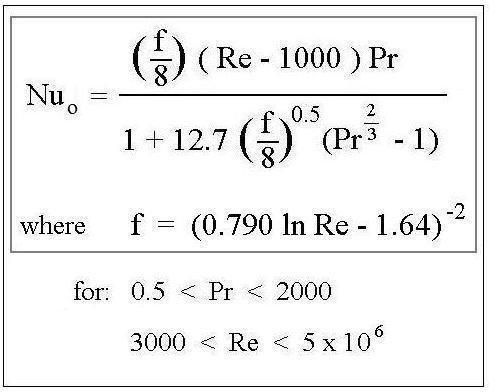but has the narrowest range of acceptable values for Re and Pr. The Dittus Boelter equation is:

Nu****o = 0.023 Re0.8Pr****0.4 , for ‘heating’ (temperature of wall > temperature of fluid), and

Nu****o = 0.026 Re0.8Pr****0.3 , for ‘cooling’ (temperature of wall < temperature of fluid).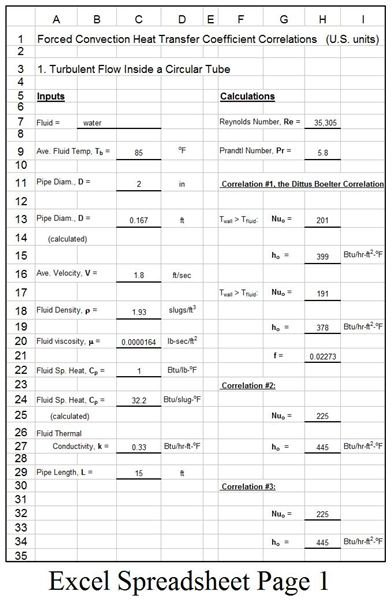Subject to: 0.7 < Pr < 120 ; 10,000 < Re < 160,000; L/D > 10 ( L/D > 50 according to some authors).

A second correlation (ref #2) is shown in the upper box at the right, along with its range of application.

A third correlation, which is a minor variation of the second one, is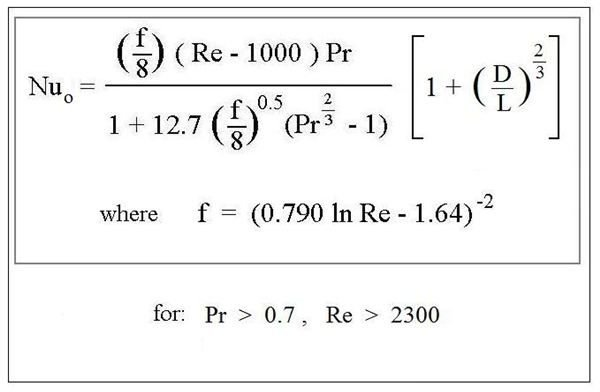described by Petukhov (ref #3). It covers a wider range of values for Re and Pr, as shown in the lower box at the right. Note that in some places f is defined in terms of log10(Re) rather than in terms of ln(Re) as follows: f = (1.82 log10(Re) - 1.64)-2. Both expressions give nearly the same value of f for a given Re value.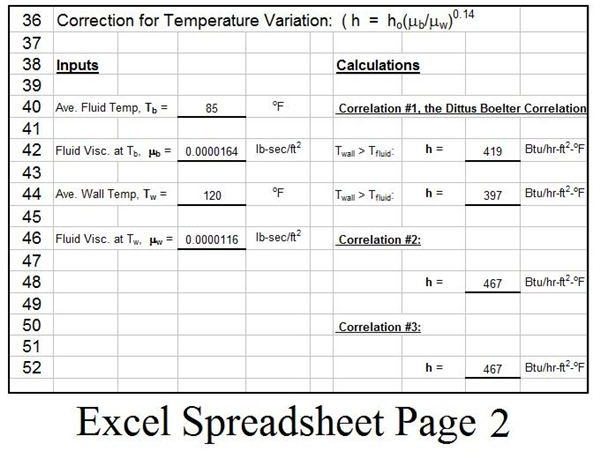Excel spreadsheets work well for calculations with these correlations. The spreadsheet template shown at the left (2 pages) has Excel formulas to calculate the Nusselt number (and then the forced convection heat transfer coefficient, h,) by all three of these correlations, based on a set of input values that allow calculation of Re and Pr, and allow calculation of h from Nu.

## Correlations/Excel Spreadsheets for Laminar Flow inside a Circular Pipe

The Excel spreadsheets in this section are all for laminar flow inside a circular pipe (Re < 2300). The equations in this section all come from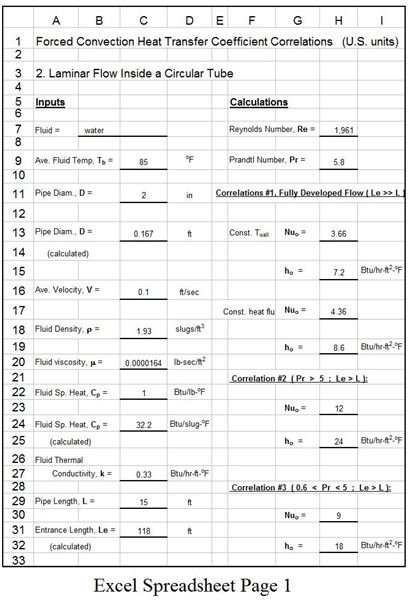Incropera et al (ref #4). The simplest case is for fully developed laminar flow. In that case the equations for Nuo are as follows:

Constant Wall Temperature: Nu****o = 3.66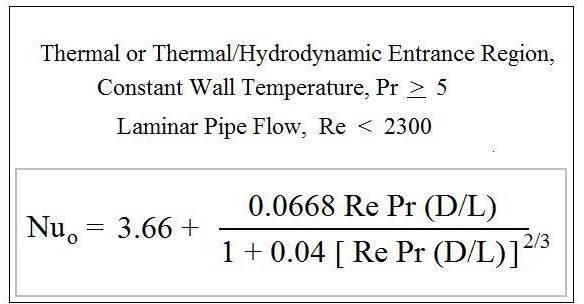Constant Heat Flux: Nu****o - 4.36

For laminar flow, the length of the entrance region, Le, can be estimated from: Le/D = 0.06*Re. If Le is quite small compared with the total pipelength, then the flow can be treated as fully developed flow. For background information about fully developed laminar flow, see the article, “Pipe Flow Calculations 1: The Entrance Length for Fully developed Flow.” If the length Le is a significant portion of the total flow, then the equations given next, for developing flow should be used.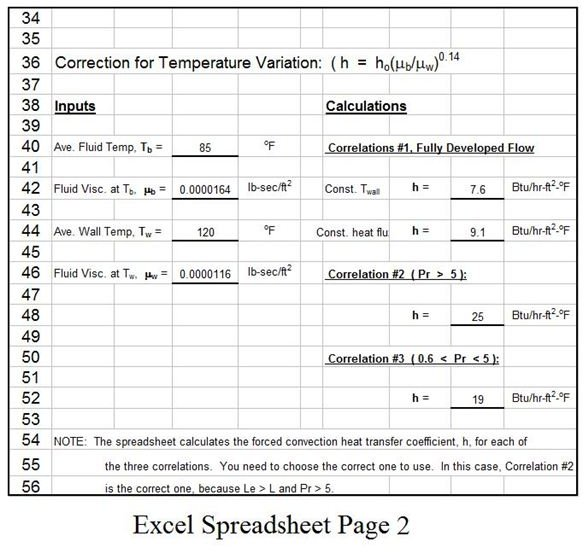For a thermal entrance region or combined thermal and hydrodynamic entrance region with Pr > 5 and constant wall temperature, the equation in the upper box at the right can be used.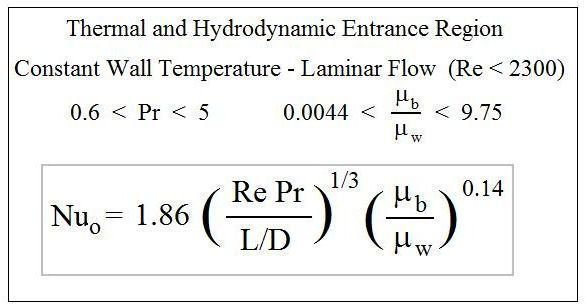For a combined thermal and hydrodynamic entrance region with constant wall temperature, lower Pr as shown, and the μb/μw condition shown, the equation in the lower box at the right can be used.

The spreadsheet template shown at the left above (2 pages) has Excel formulas to calculate the Nusselt number (and then the forced convection heat transfer coefficient, h,) by all three of these correlations, based on a set of input values that allow calculation of Re and Pr, and allow calculation of h from Nu.

Editor’s Note: We have been told the spreadsheet with U.S. units contained an error. We have corrected the error and the updated spreadsheet is available by clicking this link.

## Correlations/Excel Spreadsheets for Turbulent Flow Inside Non-circular Ducts

A general approach for turbulent flow in a non-circular duct is to use the hydraulic diameter, Dh, in place of the diameter, D, in any of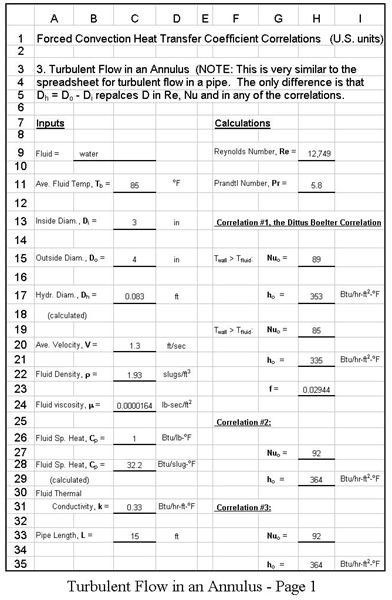the correlations for turbulent flow in a circular pipe. The hydraulic diameter is defined as follows:

Dh = 4 A/P, where A is the cross-sectional area of flow and P is the wetted perimeter.

The particular non-circular cross-section, the annulus, is of interest for applications like the double pipe heat exchanger. For flow through an annular duct with inner diameter = Di and outer diameter = Do, the hydraulic diameter is:

Dh = 4 A/P = 4[ (π/4(Do2 - Di2))/(π(Do + Di), which simplifies to: D****h = D****o - D****i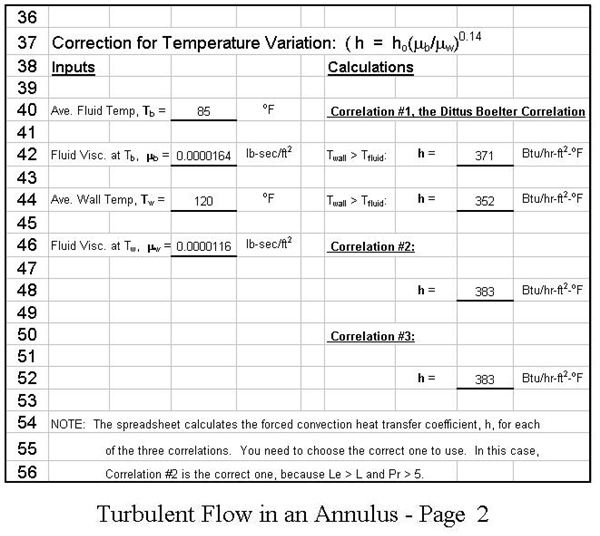The spreadsheet template shown in the two images at the right is set up to calculate Nuo, ho, and h for turbulent flow through an annulus. The Excel formulas in this spreadsheet use the three correlations used above for turbulent flow inside a circular pipe. The only difference is use of Dh ( = Do - Di) in place of D in Re, Nu, and the correlations.

## Correlations/Excel Spreadsheets for Flow across a Circular Cylinder or a Bank of Tubes

For flow across a circular cylinder or across a bank of tubes, the following definitions apply to the Reynolds number and Nusselt number:

Re****λ = λVρ/μ, Nu****λ = hλ/k, and λ = πD/2, where:

D = cylinder diameter; V = free stream fluid velocity; and all fluid properties are at the bulk mean fluid temperature [ (Tin + Tout)/2 ].

Following is a correlation (Gnielinski, Ref #5) for flow past a single circular cylinder:

Nu****λo = 0.3 + ( (Nuλ lam)2 **+ (Nuλ turb)**2 **)**1/2, where

Nu****λ lam = 0.664(Reλ)1/2(Pr)1/3, and **Nu**λ turb = (0.037 Reλ0.8 Pr)/[ 1 + 2.44 Reλ-0.1 (Pr****2/3 - 1) ]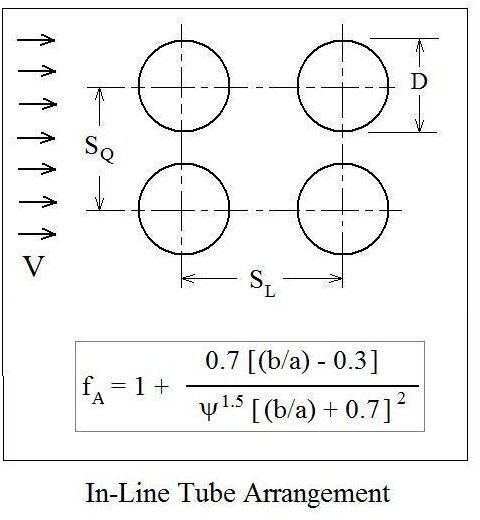This correlation is valid for 10 < Reλ < 107 and 0.6 < Pr < 1000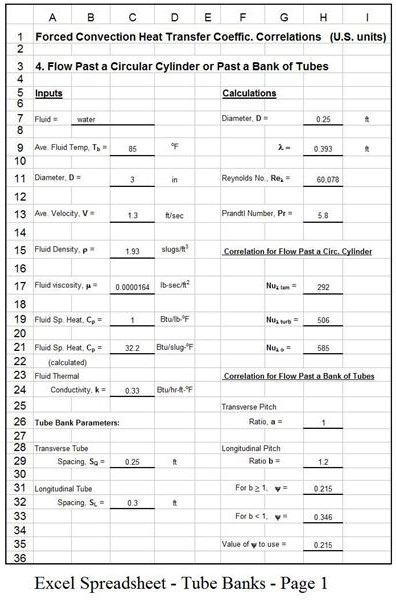Flow past a bank of tubes approximates the flow pattern in the shell side of a shell and tube heat exchanger with baffles. For flow past a bank of tubes, the correlations above are used with some modification, but additional definitions are needed as follows:

Transverse pitch ratio: a = SQ/D

Longitudinal pitch ratio: b = SL/D (SQ and SL are shown in the diagrams at the right.)

Void ratio: ψ = 1 - π/(4a), for b > 1 ; ψ = 1 - π/(4ab), for b < 1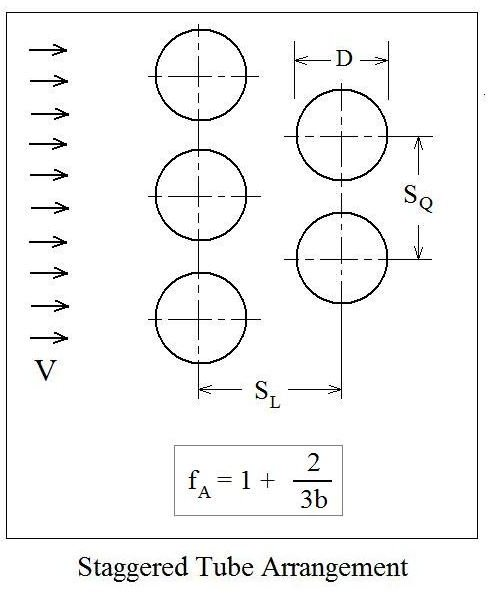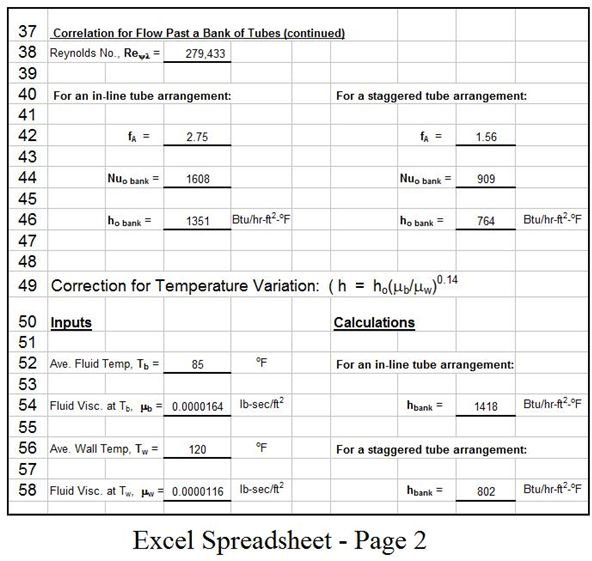The Nusselt number for a tube bank is then: Nu****o bank = f****A Nu****λo with Nuλo calculated with the equation for flow past a single cylinder given above, with Reλ replaced by Re****ψλ = λVρ/ψμ (Gnielinski, Ref #5).

The equations for fA for in-line and staggered tube banks are included in the diagrams at the right.

The spreadsheet template shown in the two images at the left above is set up to calculate Nuλo, ho, and h for flow past a single cylinder and flow past a bank of tubes. The Excel formulas in this spreadsheet use the correlations and equations given in this section.

1. Dittus, P.W. and Boelter, L.M., Univ. Calif. Pub. Eng., Vol. 1, No. 13, pp 443-461 (reprinted in Int. Comm. Heat Mass Transfer, Vol. 12, pp 3-22 (1985).

3. Petukhov, B.S., “Heat transfer and friction in turbulent pipe flow with variable physical properties,” Adv. Heat Transfer 6, 503-565 (1970).

4. Incropera, F.P., DeWitt, D.P, Bergman, T.L., & Lavine, A.S., Fundamentals of Heat and Mass Transfer, 6th Ed., Hoboken, NJ, John Wiley & Sons, (2007).

5. Gnielinski, V., “Berechnung mittlerer Warme- und Stoffubergshoeffizienten an laminar und turbulent uberstromten Einzellkorpern mit Hilfe einer einheitlichen Gleichung,” Forschung im Ingenierwesen, Vol. 41, pp 145-153, (1975).

Sample contents.

## This post is part of the series: Heat Transfer Fundamentals

Learn about conduction, convection, and radiation, the three mechanisms for heat transfer, including example calculations. Conduction heat transfer is of interest mostly in solids. Convection heat transfer is due to a moving fluid. Heat can also be transferred by electromagnetic radiation.